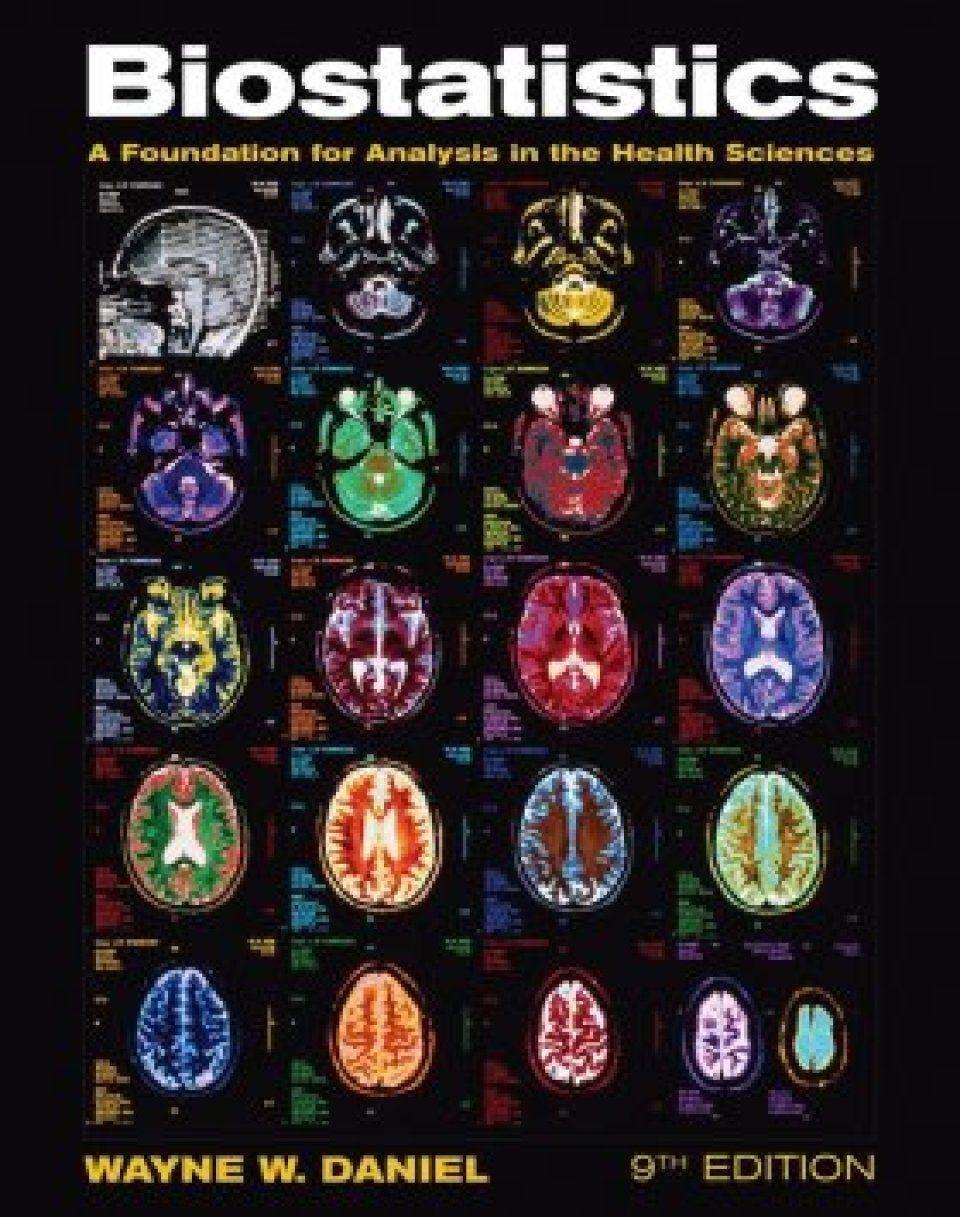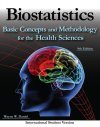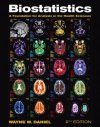## British Wildlife

8 issues per year 84 pages per issue Subscription only

British Wildlife is the leading natural history magazine in the UK, providing essential reading for both enthusiast and professional naturalists and wildlife conservationists. Published eight times a year, British Wildlife bridges the gap between popular writing and scientific literature through a combination of long-form articles, regular columns and reports, book reviews and letters.

Subscriptions from £40 per year

## Conservation Land Management

4 issues per year 44 pages per issue Subscription only

Conservation Land Management (CLM) is a quarterly magazine that is widely regarded as essential reading for all who are involved in land management for nature conservation, across the British Isles. CLM includes long-form articles, events listings, publication reviews, new product information and updates, reports of conferences and letters.

Subscriptions from £18 per year
• Huge product rangeOver 140,000 books & equipment products

• Rapid shippingUK & Worldwide

• Pay in £, € or U.S.\$By card, cheque, transfer, draft

• Exceptional customer serviceGet specialist help and advice

# Biostatistics: A Foundation for Analysis in the Health Sciences

Out of Print
By: Wayne W Daniel
960 pages, Figs, tabsClick to have a closer look
Select version
•ISBN: 9780470413333 Edition: 9 Paperback Mar 2009 Out of Print #179551
•ISBN: 9780470105825 Edition: 9 Hardback Dec 2008 Out of Print #176918

Allied health professionals rely on Biostatistics for its high standards of statistical accuracy. It helps them develop a set of statistical tools that are relevant to their field. Now in its ninth edition, the book integrates new applications from several biological science fields throughout the pages. Each chapter now opens with bulleted objectives that highlight the main ideas. Summary boxes of formulae and statistical rules are presented for easy reference and review. Support is also provided for multiple programs such as SPSS, SAS, and STATA, in addition to Minitab. This includes screen captures and technology boxes with step-by-step help. Health professionals will then gain the ability to use technology to analyze data.

## Contents

Preface. 1. Introduction To Biostatistics. 1.1 Introduction. 1.2 Some Basic Concepts. 1.3 Measurement and Measurement Scales. 1.4 Sampling and Statistical Inference. 1.5 The Scientific Method and the Design of Experiments. 1.6 Computers and Biostatistical Analysis. 1.7 Summary. Review Questions and Exercises. References. 2. Descriptive Statistics. 2.1 Introduction. 2.2 The Ordered Array. 2.3 Grouped Data: The Frequency Distribution. 2.4 Descriptive Statistics: Measures of Central Tendency. 2.5 Descriptive Statistics: Measures of Dispersion. 2.6 Summary. Review Questions and Exercises. References. 3. Some Basic Probability Concepts. 3.1 Introduction. 3.2 Two Views of Probability: Objective and Subjective. 3.3 Elementary Properties of Probability. 3.4 Calculating the Probability of an Event. 3.5 Bayes' Theorem, Screening Tests, Sensitivity, Specificity, and Predictive Value Positive and Negative. Summary. Review Questions and Exercises. References. 4. Probability Distributions. 4.1 Introduction. 4.2 Probability Distributions of Discrete Variables. 4.3 The Binomial Distribution. 4.4 The Poisson Distribution. 4.5 Continuous Probability Distributions. 4.6 The Normal Distribution. 4.7 Normal Distribution Applications. 4.8 Summary. Review Questions and Exercises. References. 5. Some Important Sampling Distributions. 5.1 Introduction. 5.2 Sampling Distributions. 5.3 Distribution of the Sample Mean. 5.4 Distribution of the Difference Between Two Sample Means. 5.5 Distribution of the Sample Proportion. 5.6 Distribution of the Difference Between Two Sample Proportions. 5.7 Summary. Review Questions and Exercises. References. 6. Estimation. 6.1 Introduction. 6.2 Confidence Interval for a Population Mean. 6.3 The t Distribution. 6.4 Confidence Interval for the Difference Between Two Population Means. 6.5 Confidence Interval for a Population Proportion. 6.6 Confidence Interval for the Difference Between Two Population Proportions. 6.7 Determination of Sample Size for Estimating Means. 6.8 Determination of Sample Size for Estimating Proportions. 6.9 Confidence Interval for the Variance of a Normally Distributed Population. 6.10 Confidence Interval for the Ratio of the Variances of Two Normally Distributed Populations. 6.11 Summary. Review Questions and Exercises. References. 7. Hypothesis Testing. 7.1 Introduction. 7.2 Hypothesis Testing: A Single Population Mean. 7.3 Hypothesis Testing: The Difference Between Two Population Means. 7.4 Paired Comparisons. 7.5 Hypothesis Testing: A Single Population Proportion. 7.6 Hypothesis Testing: The Difference Between Two Population Proportions. 7.7 Hypothesis Testing: A Single Population Variance. 7.8 Hypothesis Testing: The Ratio of Two Population Variances. 7.9 The Type II Error and the Power of a Test. 7.10 Determining Sample Size to Control Type II Errors. 7.11 Summary. Review Questions and Exercises. References. 8. Analysis Of Variance. 8.1 Introduction. 8.2 The Completely Randomized Design. 8.3 The Randomized Complete Block Design. 8.4 The Repeated Measures Design. 8.5 The Factorial Experiment. 8.6 Summary. Review Questions and Exercises. References. 9. Simple Linear Regression And Correlation. 9.1 Introduction. 9.2 The Regression Model. 9.3 The Sample Regression Equation. 9.4 Evaluating the Regression Equation. 9.5 Using the Regression Equation. 9.6 The Correlation Model. 9.7 The Correlation Coefficient. 9.8 Some Precautions. 9.9 Summary. Review Questions and Exercises. References. 10. Multiple Regression And Correlation. 10.1 Introduction. 10.2 The Multiple Linear Regression Model. 10.3 Obtaining the Multiple Regression Equation. 10.4 Evaluating the Multiple Regression Equation. 10.5 Using the Multiple Regression Equation. 10.6 The Multiple Correlation Model. 10.7 Summary. Review Questions and Exercises. References. 11. Regression Analysis: Some Additional Techniques. 11.1 Introduction. 11.2 Qualitative Independent Variables. 11.3 Variable Selection Procedures. 11.4 Logistic Regression. 11.5 Summary. Review Questions and Exercises. References. 12. The Chi-Square Distribution And The Analysis Of Frequencies. 12.1 Introduction. 12.2 The Mathematical Properties of the Chi-Square Distribution. 12.3 Tests of Goodness-of-Fit. 12.4 Tests of Independence. 12.5 Tests of Homogeneity. 12.6 The Fisher Exact Test. 12.7 Relative Risk, Odds Ratio, and the Mantel-Haenszel Statistic. 12.8 Survival Analysis. 12.9 Summary. Review Questions and Exercises. References. 13. Nonparametric And Distribution-Free Statistics. 13.1 Introduction. 13.2 Measurement Scales. 13.3 The Sign Test. 13.4 The Wilcoxon Signed-Rank Test for Location. 13.5 The Median Test. 13.6 The Mann-Whitney Test. 13.7 The Kolmogorov-Smirnov Goodness-of-Fit Test. 13.8 The Kruskal-Wallis One-Way Analysis of Variance by Ranks. 13.9 The Friedman Two-Way Analysis of Variance by Ranks. 13.10 The Spearman Rank Correlation Coefficient. 13.11 Nonparametric Regression Analysis. 13.12 Summary. Review Questions and Exercises. References. 14. Vital Statistics. 14.1 Introduction. 14.2 Death Rates and Ratios. 14.3 Measures of Fertility. 14.4 Measures of Morbidity. 14.5 Summary. Review Questions and Exercises. References. Appendix. Statistical Tables. Answers To Odd-Numbered Exercises. Index.

## Biography

Wayne W. Daniel is on the faculty of Georgia State University, Atlanta, Georgia.

Out of Print
By: Wayne W Daniel
960 pages, Figs, tabs